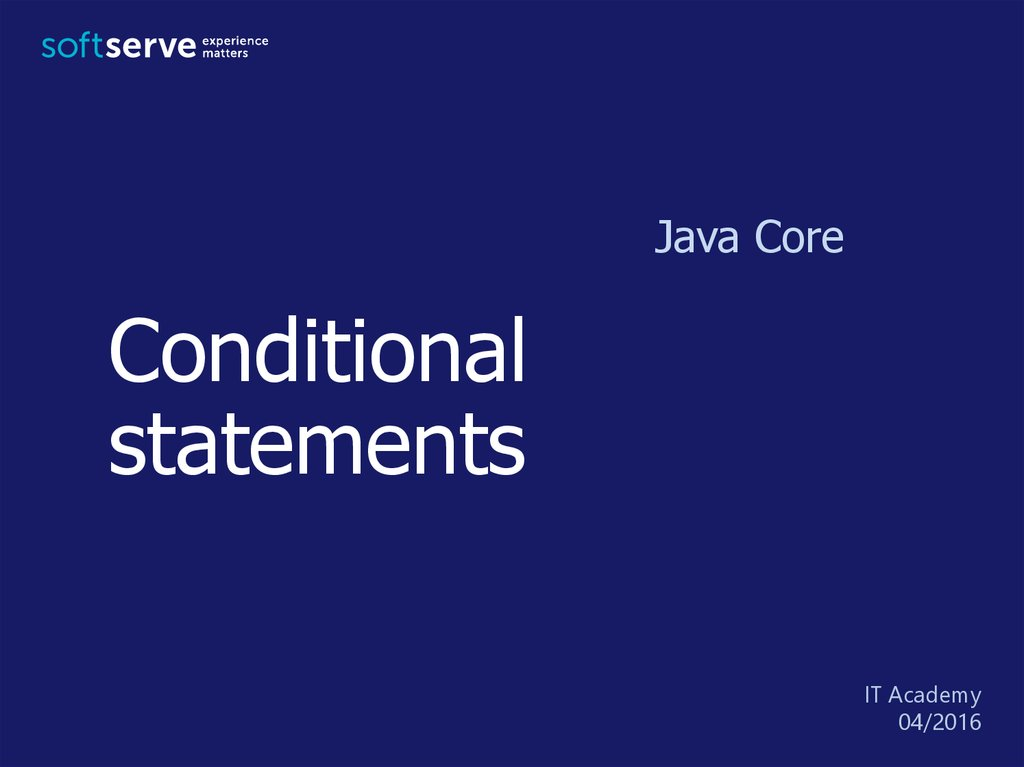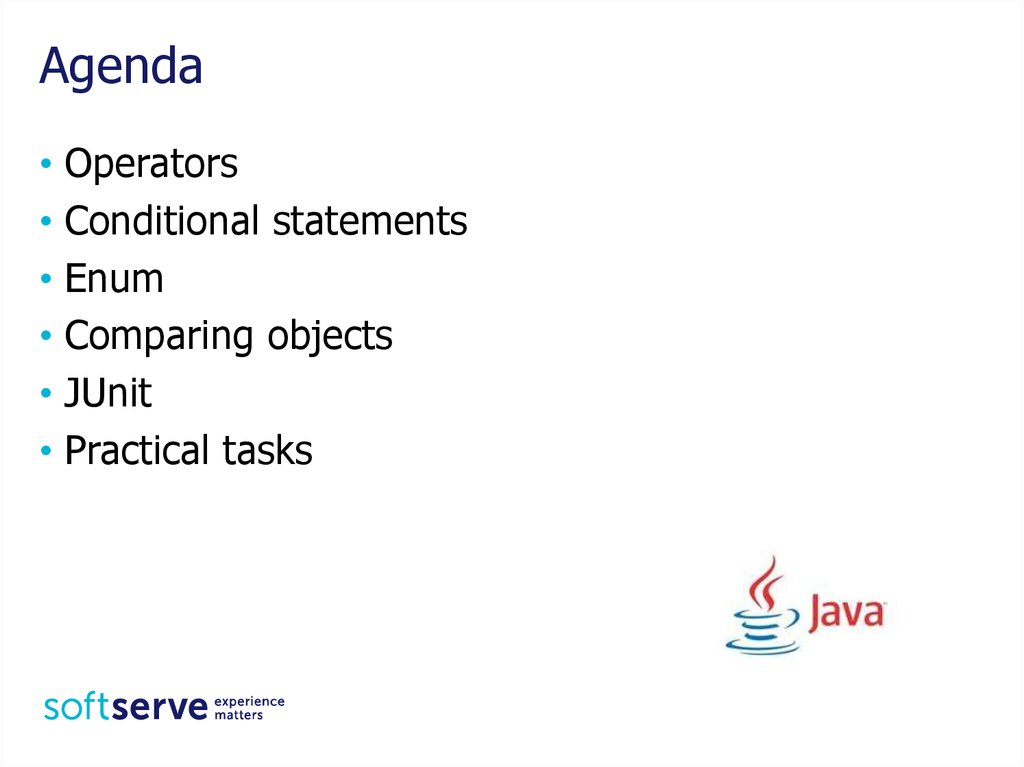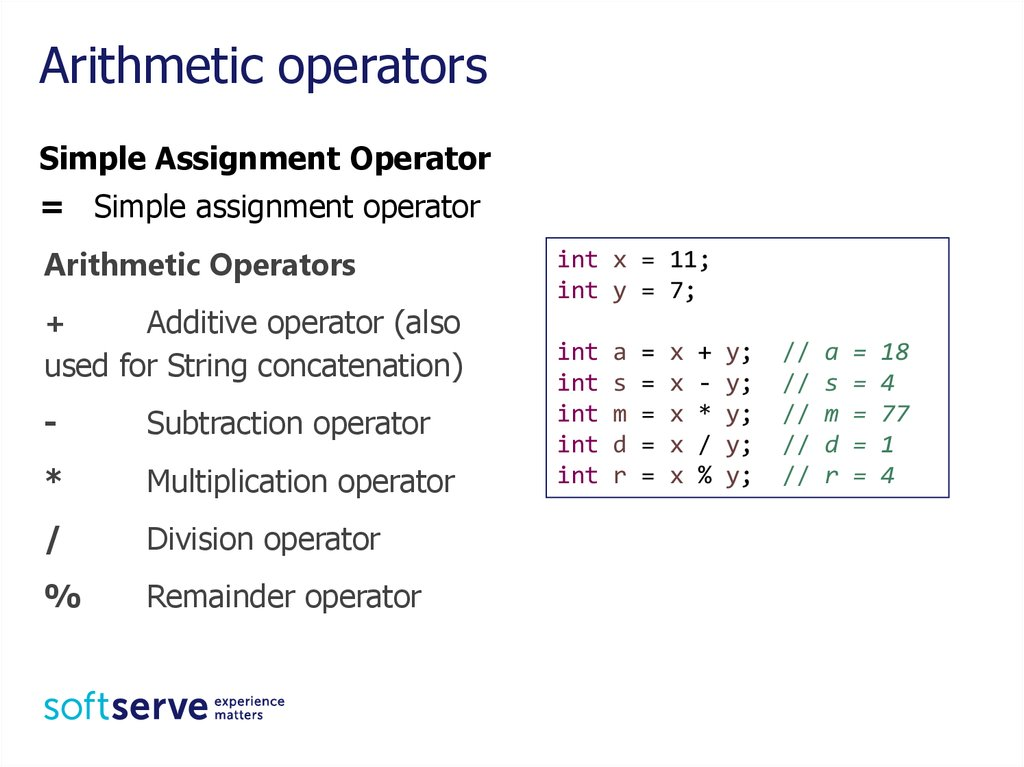# Conditional statements. Java Core

Java Core
Conditional
statements
04/2016

## 2. Agenda

• Operators
• Conditional statements
• Enum
• Comparing objects
• JUnit

## 3. Arithmetic operators

Simple Assignment Operator
= Simple assignment operator
Arithmetic Operators
+
used for String concatenation)
-
Subtraction operator
*
Multiplication operator
/
Division operator
%
Remainder operator
int x = 11;
int y = 7;
int
int
int
int
int
a
s
m
d
r
=
=
=
=
=
x
x
x
x
x
+
*
/
%
y;
y;
y;
y;
y;
//
//
//
//
//
a
s
m
d
r
=
=
=
=
=
18
4
77
1
4

## 4. Unary Operators

Unary Operators
+
Unary plus; indicates
positive value
Unary minus; negates an
expression
++ Increment operator;
increments a value by 1
-- Decrement operator;
decrements a value by 1
!
Logical complement
operator; inverts the value
of a boolean
int x = 5;
int a, b;
a = x++;
// a = 5 x = 6
x--;
// x = 5
b = ++x;
// b = 6 x = 6
++x;
// x = 7
boolean bool = true;
// true
System.out.println(bool);
// false
System.out.println(!bool);

## 5. Equality and Relational Operators

Equality and Relational Operators
==
Equal to
!=
>
>=
Not equal to
Greater than
Greater than or equal to
<
<=
Less than
Less than or equal to
int x = 5;
int y = -5;
System.out.println(x == y); // false
System.out.println(x != y); // true
System.out.println(x >= y); // true

## 6. Conditional Operators

The && and || operators perform Conditional-AND and
Conditional-OR operations on two boolean expressions.
&& Conditional-AND
||
Conditional-OR
score >= 3 && score <= 5
day == "Saturday" || day == "Sunday"
What is the result?
int t = 5, s = 4, v = 7;
System.out.println(t > s && t > v || s < v);
System.out.println((t < v || s > v) && t < s);

## 7. Ternary Operator ? :

Ternary (conditional operator) consists of three
operands and is used to evaluate Boolean
expressions. The goal of the operator is to
decide which value should be assigned to the
variable.
condition ? value1 : value2
What are the values of variable str1 and str2?
int t = 5, s = 4;
String str1 = t >= ++s? "yes" : "no";
int a = 3, b = 2;
String str2 = a-- == b ? "yes" : "no";

## 8. Statement if

The if-then statement tells your program to execute a
certain section of code only if a particular test
evaluates to true.
if (condition) {
then-statement;
}
if (condition) {
then-statement;
} else {
else-statement;
}
if (temperature<10) {
System.out.println(“It’s too cold”);
} else {
System.out.println(“It’s Ok”); ;
}

## 9. switch

A switch statement allows a variable to be tested for equality
against a list of values. Each value is called a case, and the variable
being switched on is checked for each case.
switch (expression)
{
case const-expr1 :
statement(s);
break;
case const-expr1 :
statement(s);
break;
default :
statement(s);
break;
}

## 10. Example

System.out.println("Do you enjoy Java? (yes/no/maybe)");
switch (input.toLowerCase()) {
case "yes":
case "maybe":
System.out.println("Great!");
break;
case "no":
break;
default:
System.out.println("Wrong!");
}

## 11. Enum

An enum type is a special data type that enables for a variable
to be a set of predefined constants. The variable must be
equal to one of the values that have been predefined for it.
public enum Season {
WINTER, SPRING, SUMMER, AUTUMN
}
Season season;
season = Season.WINTER;

## 12. Example

Season season;
...
switch (month) {
case "Desember": case "January": case "February":
season = Season.WINTER; break;
case "Marth": case "April": case "May":
season = Season.SPRING; break;
case "June": case "Jule": case "August":
season = Season.SUMMER; break;
case "September": case "October": case "November":
season = Season.AUTUMN; break;
default:
System.out.println("No this month");
System.exit(0);
}

## 13. Comparing objects

public class Student {
private String name;
private int age;
}
public Student(String name, int age) {
this.name = name;
this.age = age;
}
getters, setters ...
Which will be the results?
Student student1 = new Student("Ira", 25);
Student student2 = new Student("Ira", 25);
System.out.println(student1 == student2);
System.out.println(student1.equals(student2));

## 14. hashCode

@Override
public int hashCode() {
final int prime = 31;
int result = 1;
result = prime * result + age;
result = prime * result
+ ((name == null) ? 0 : name.hashCode());
return result;
}

## 15. equals

@Override
public boolean equals(Object obj) {
if (this == obj) return true;
if (obj == null) return false;
if (getClass() != obj.getClass()) return false;
Student other = (Student) obj;
if (age != other.age) return false;
if (name == null) {
if (other.name != null) return false;
} else if (!name.equals(other.name)) return false;
return true;
}
Which will be the results?
Student student1 = new Student("Ira", 25);
Student student2 = new Student("Ira", 25);
System.out.println(student1 == student2);
System.out.println(student1.equals(student2));

## 16. JUnit Framework

JUnit is a unit testing framework for the Java
programming language.
JUnit has been important in the development of
test-driven development, and is one of a family of
unit testing frameworks collectively known as xUnit
that originated with SUnit.

## 17. Testing Problems

• Programmers should write tests
• As you probably know programmers always busy,
and they have no time to write tests
• They need some tool that can help them
• Main requirements for this tool:
• A few lines of code then test should run
• To write test that won’t run, then write the code that
will make run

## 18. JUnit plugin for Eclipse IDE

• Mr. Erich Gamma who is a one of developers of
JUnit framework also known as a Eclipse IDE
developer
• JUnit well integrated into Eclipse IDE

## 19. JUnit assertion methods

Method
Description
assertEquals()
See if two objects or primitives have the
same value
assertNotEquals()
assertSame()
See if two objects are the same object
assertNotSame()
assertTrue()
Test a Boolean expression
assertFalse()
assertNull()
assertNotNull()
Test for a null object

JUnit

JUnit

## 22. Class Calc

public class Calc {
public int add(int a, int b) {
return a + b;
}
public int div(int a, int b){
return a / b;
}
}

## 23. Class CalcTest

import static org.junit.Assert.*;
import org.junit.Test;
public class CalcTest {
Calc calc = new Calc();
@Test
@Test
public void testDivPositive() {
int actual = 4;
int expected = calc.div(9, 2);
assertEquals(actual, expected);
}
@Test(expected = Exception.class)
public void testDivZero() {
int actual = calc.div(23, 0);
}
}

1. Enter three numbers. Find out how many of them are odd.
2. Enter the number of the day of the week. Display the
name in three languages.
3. Enter the name of the country. Print the name of the
continent. (Declare enum with names of continents)
4. Create class Product with fields name, price and quantity.
Create four instances of type Product.
Display the name and quantity of the most expensive item.
Display the name of the items, which has the biggest
quantity.

## 25. HomeWork (online course)

• UDEMY course "Java
Tutorial for Complete
Beginners":
https://www.udemy.com
/java-tutorial/
• Complete lessons 10,
11, 13:

## 26. Unit Testing with JUnit

• Short step-by-step online course:
https://www.udemy.com/junit-tutorial-for-beginners-with-javaexamples/learn/v4/overview

## 27. Homework

a) read 3 float numbers and check: are they all belong to the
range [-5,5];
b) read 3 integer numbers and write max and min of them;
c) read number of HTTP Error (400, 401,402, ...) and write the
name of this error (Declare enum HTTPError)
2. Сreate class Dog with fields name, breed, age.
a) Declare enum for field breed.
b) Create 3 instances of type Dog.
c) Check if there is no two dogs with the same name.
d) Display the name and the kind of the oldest dog.

## 28. The end

USA HQ
Toll Free: 866-687-3588
Tel: +1-512-516-8880
Ukraine HQ
Tel: +380-32-240-9090
Bulgaria
Tel: +359-2-902-3760
Poland
Tel: +48-71-382-2800
EMAIL
[email protected]
Germany
Tel: +49-69-2602-5857
UK
Tel: +44-207-544-8414
WEBSITE:
www.softserveinc.com
Netherlands
Tel: +31-20-262-33-23